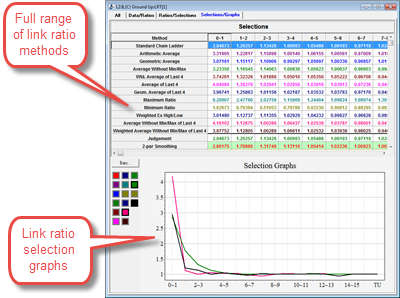# ELRF™ Enterprise: Actuarial Reserving Software

### Request ELRF™ demonstration

A full ELRF™ actuarial software presentation takes around 1 hour.

Demonstrations can be arranged either on-site or online. Submit your contact information and an Insureware representative will be in touch.

### Extended Link Ratio Family (ELRF) modeling framework

The Extended Link Ratio Family (ELRF) modeling framework formulates average link ratio methods as regression estimators and extends them. This includes Mack (volume weighted averages), other weighted averages and extensions:

• Murphy (an intercept)
• A constant trend in the incrementals for each development period down the accident years
• Optimal combinations of intercepts, trends, and link ratios
• Control over variance assumptions (delta)

Standard deviations are computed analytically in all cases.

### The bootstrap technique

The Bootstrap technique in the ELRF module gives

• Distributions of reserves by accident years, calendar years, and total
• Percentiles, V@Rs and T-V@Rs
• … and more!

Distributions can be translated from the bootstrap sample mean to any mean specified by the user by accident period totals or calendar period totals. This allows the actuarial analyst to fit any link ratio model, obtain the means by accident (or calendar) period and apply the bootstrap using the Mack method residuals with a selected delta.

### Diagnostic statistics and graphs

Diagnostic statistics to test assumptions made by any ELRF model

• Residuals versus development year, accident year, calendar year, and fitted
• Y|X plots to test whether link ratios have predictive power in the presence of an intercept
• … and more!

The regression formulation of link ratios (Mack, Murphy, and other extensions), provides the framework for verifying the assumptions made by the link ratio techniques.

### Link ratios, Mack, Murphy, Over-Dispersed Poisson, and the bootstrap techniqueDo link ratio methods work for your data? Introduction to link ratio methods Link ratio methods formulated as regression estimators The Mack (Chain Ladder) and Murphy methods The Extended Link Ratio Family (ELRF) The bootstrap technique Link ratio methods and correlation Comparison with the optimal model in the PTF modeling framework Real life case studies! Read more on the ELRF™ modeling framework.

### Link Ratio Techniques (LRT) moduleThe LRT module include a full range of link ratio methods:

• Chain Ladder (Volume weighted averages);
• Arithmetic Average;
• Geometric Average;
• Average without min/max;
• Wtd. Average of last N*;
• Average of Last N*;
• Geom. Average of Last N*;
• Maximum Ratio
• Minimum Ratio
• Weighted Ex high/low;
• Two and three parameter smoothing;
• Bornhuetter-Ferguson;
• and more!

*N is configurable by the analyst.

ELRF Enterprise provides the complete actuarial reserving software solutions for actuaries wanting to test and validate link ratio methodology.